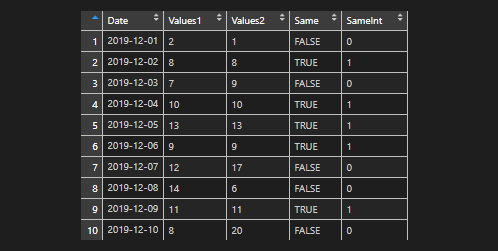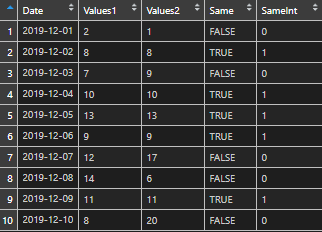# Convert R TRUE and FALSE values to 1 and 0, and vice versaHere is a simple method to convert R TRUE and FALSE values to 1 and 0, and vice versa.

Here is my data frame.

If I’m doing some calculation that gives me TRUE or FALSE then sometimes it is necessary to convert them to 1 and 0.The easiest way is by using a function as.integer.## Convert R 1 and 0 values to TRUE and FALSE

Sometimes it’s another way around. Here you go.

If you want to know how to reflow your code or other useful RStudio tips and tricks, take a look at this post.